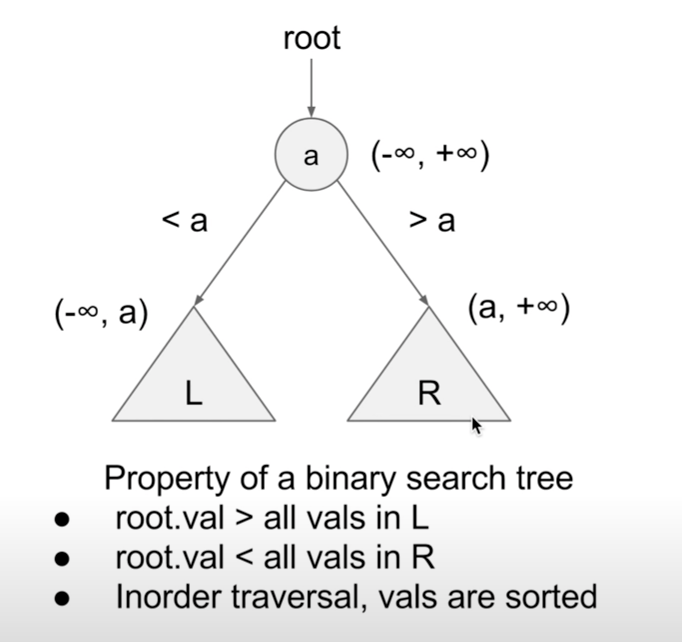# 98.Validate Binary Search Tree

September 30, 2021

### Description:

Given the root of a binary tree, determine if it is a valid binary search tree (BST).

A valid BST is defined as follows:

• The left subtree of a node contains only nodes with keys less than the node’s key.
• The right subtree of a node contains only nodes with keys greater than the node’s key.
• Both the left and right subtrees must also be binary search trees.

### Constraints:

• The number of nodes in the tree is in the range [1, 104].
• -2^31 <= Node.val <= 2^31 - 1

### Note:

#### Property of a Binary Search Tree:For each TreeNode

• cur->left->val < cur->val
• cur->right->val > cur->val

#### Consider the range:

The input value ranges from -2^31 <= Node.val <= 2^31 - 1, so the positive/negative infinity should be set to LLONG_MIN, LLONG_MAX. (64-bit)

### C++:

#### For Your Consider:

What if the range extends to -2^64 ~2^64-1?

Hint: Use nullptr to represent infinity.